﻿ Comparison of Geodesics and Energy Variation Principles of Light Propagation

### Comparison of Geodesics and Energy Variation Principles of Light PropagationOPEN ACCESSPEER-REVIEWED

## Comparison of Geodesics and Energy Variation Principles of Light Propagation

Wladimir BelayevCenter for Relativity and Astrophysics, Saint-Petersburg, Russia

### Abstract

The propagation of light on a set of null paths in a pseudo-Riemannian manifold is studied using a variational principle of extremal energy. This method is compared with those based on the geodesics and Fermat principles. For the static space-time all three variational methods give the same solutions. The Fermat principle for stationary gravitational fields is identical to the principle of variation of energy of light-like particles. The approach proposed here is applied to a flat expanding space, corresponding to the LCDM-model, and generalized Gödel space-time. In the first case a solution obtained by the method of energy variation coincides with the geodesic method, while in the second case they give different solutions.

• Belayev, Wladimir. "Comparison of Geodesics and Energy Variation Principles of Light Propagation." International Journal of Physics 1.1 (2013): 1-4.
• Belayev, W. (2013). Comparison of Geodesics and Energy Variation Principles of Light Propagation. International Journal of Physics, 1(1), 1-4.
• Belayev, Wladimir. "Comparison of Geodesics and Energy Variation Principles of Light Propagation." International Journal of Physics 1, no. 1 (2013): 1-4.

 Import into BibTeX Import into EndNote Import into RefMan Import into RefWorks

### 1. Introduction

In general relativity light is assumed to propagate in the vacuum along null geodesics in a pseudo-Riemannian manifold. Geodesic equations can be obtained using a variational method for the integral of motion. In a general case the geodesic is extremal in a set that includes isotropic and non-isotropic curves with close to zero length of space-time path . Besides the geodesics principle there exists Fermat principle for stationary gravity fields. In  a variational method without the violation of isotropy of the path of the light-like particle is proposed and it is shown that it gives equations identical to those which follow from the Fermat principle. For the Gödel space these methods give solutions being different from the null geodesic.

In the present paper the class of metrics extended by metrics with zero coefficientcorresponding to the time coordinate is considered. We also find a solution by the proposed method of energy variation for a generalized Gödel space and compare it with that obtained in .

Our results here lead us to a search to find additional criteria for the choice between the null geodesic principle and the variational method without violation of isotropy of the path.

### 2. Fermat and Geodesics Principles

For a stationary spacetime with appropriate metric tensorthe Fermat principle is formulated  as follows(1)

whereis the first variation andis element of spatial distance along the ray:(2)

Here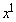is the time coordinate and indicesare the space coordinates.

The null geodesics equations with affine parameterand the four-velocity vectorare written as(3)

where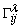are Christoffel symbols.

For a static spacetime the Fermat principle and the geodesics equations coupled with equation of null geodesic condition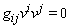(4)

give the same solutions .

### 3. Lagrangian for a Light-Like Particle

In the principle of energy variation for a light-like particle’s motion, the pseudo-Riemannian metric with coefficients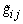defined by a transformation(5)

is written in form(6)

whereis some quantity, which is assumed to be equal 1 and regarded as the energy of the light-like particle with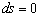. Solving this equation forunder conditiongives two solutions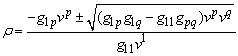(7)

Even if one solution in accordance with making definitions will be.

With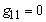and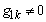even if for one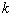the energy takes form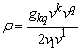(8)

In both cases for the free moving particle the lagrangian is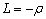(9)

Its derivatives give the canonical momenta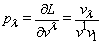(10)

and the forces(11)

### 4. Equations of Motion Produced by Energy Variation and Fermat Principles

In energy variation principle equations of motion are found from the principle of action(12)

where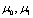, are values of the affine parameter of the points, which are linked by trajectory of particle. Taking into account (9), variation of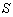leaves interval to be light-like, and an application of standard variational procedure yields Euler-Lagrange equations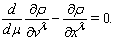(13)

Withwe obtain(14)

For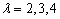the Euler-Lagrange equations give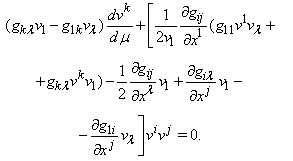(15)

Let’s show that for the stationary spacetime the above equations are identical to those which follow from the Fermat principle. Denoting(16)

and comparing this expression with (7) we write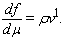(17)

Therefore, variation (1) is equivalent to variation of integral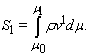(18)

The Euler-Lagrange equation, corresponded to the time coordinate, will be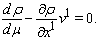(19)

Since the energyis assumed to be constant along critical curve, its differential is zero. The metrical coefficients in case of the stationary field doesn't depend on time, therefore we have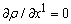. Thus, expression (19) is idential to equation (14). For the space coordinates, the equations are follows: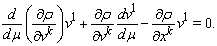(20)

We condition by appropriate choice of the affine parameterthe constant value of. Then, the second term in the left part of equations will be vanishing and they shall be identical to (13).

### 5. Flat Expanding Space

Let's find by the proposed method the extremal isotropic curves in the flat expanding space, which corresponds to thecosmological model. With rectangular coordinates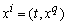it is described by metric(21)

where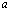is the length scale factor.

Equation (14) gives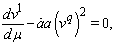(22)

where overdot denotes derivative with respect to time. The Euler-Lagrange equations for the cyclic coordinates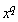yield constants of motion(23)

Extracting the derivatives with respect to the space-like coordinates from this equation and substituting them in (22) we obtain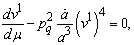(24)

This equation has a solution, which with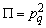is written in form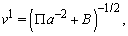(25)

whereis constant. Substitutionin equation (23) gives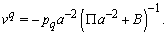(26)

The condition (4) will be(27)

It yieldsand components of the four-velocity vector turn out to(28)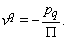(29)

They conform to solution of equations of the null geodesics in FRLW spacetime .

### 6. Generalized Gödel Space-Time

The stationary solution of the Einstein field equation with cosmological constant found by Gödel describes gravity field of the rotating uniform dust matter. With coordinatesthe line element for generalized Gödel metric is written in form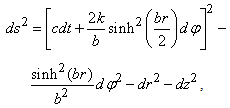(30)

whereare constants.

The canonical momenta (10) for cyclic coordinates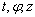are the constants of motion. They are written in form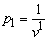(31)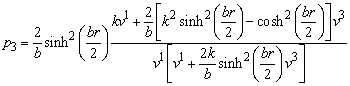(32)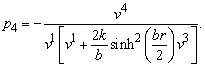(33)

These equations with condition of null geodesic(34)

yield components of the four-velocity vector: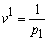(35)(36)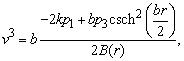(37)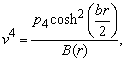(38)

where function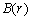is(39)

The canonical momenta must satisfy the condition(40)

since only in this case the radial velocity exists. The singularity takes place with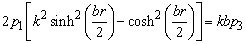(41)

Let's compare four-velocity obtained above with solution of the null geodesics equations . For the affine parameter, being proportional to the coordinate time with corresponding constants, the null geodesics equations yield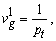(42)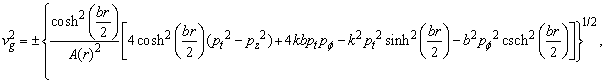(43)(44)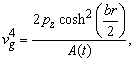(45)

where functionis(46)

Though with(47)

both methods give identical space curve, the coordinate velocity along it will be different.

### 7. Conclusions

For the static spacetime all three variational methods give the same solutions, also Fermat principle for stationary gravity fields is identical to the principle of variation of energy of light-like particles. Though expression for energy is different in cases of zero and non-zero metrical coefficients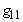that does not influence the form of the canonical momenta and forces as well as the expressions for equations of motion. For the flat expanding space, a curve corresponding to the critical value of integral of the photon energy coincides with geodesic, while for the generalized Gödel space-time these solutions are different.

Only experiment can establish which of the principles, geodesics principle or energy variation, conforms better to physical reality. If it will be demonstrated experimentally that with some physical conditions a motion of material test particle corresponds to the spacetime, for which these methods give different solutions for the light, then experimental determination of the light trajectory in the same physical conditions allows to make choice between these principles.

### Acknowledgement

I am grateful to Vish Viswanathan for help in preparing of this manuscript.

### References

  Belayev, W.B., “Application of Lagrange mechanics for analysis of the light-like particle motion in pseudo-Riemann space”, Int. J. Th. and Math. Phys. 2(2): 10-15. Apr. 2012.In article  Dautcourt G., “The lightcone of Gödel-like spacetimes”, Class.Quant.Grav. 27(22). 225024. Nov. 2010, arXiv:1009.5231.In article  Landau L.D. and Lifshitz E.M., Classical Theory of Fields, Fourth Revised English Edition, Butterworth–Heinemann, Oxford, 273, 1975.In article  Pauli W, Theory of relativity, Pergamon press, New York, 1958.In article  McVittie G.C, General Relativity and Cosmology, London, Chapman and Hall Ltd., 1956.In article